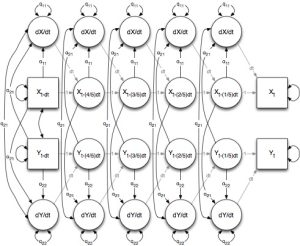# Integration of Stochastic Differential Equations Using Structural Equation Modeling (2016)

These are the appendices associated with the article: Deboeck, P. R. & Boulton, A. J. (2016).  Integration of Stochastic Differential Equations Using Structural Equation Modeling: A Method to Facilitate Model Fitting and Pedagogy. Structural Equation Modeling.

AppendicesInSDE.zip